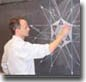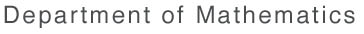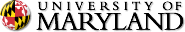This page is not longer being maintained. Please visit the new UMD Mathematics website at www-math.umd.edu.Math Home > Research > Seminars > Numerical Analysis Seminar > [ Search | Contact | Help! ]

# NUMERICAL ANALYSIS SEMINAR

### Department of Mathematics, University of Maryland, College Park

```
S P R I N G   1 9 9 4   S C H E D U L E

-----------------------------------------------------------------------------
Jan. 27:  A level set approach to computing solutions to incompressible
two-fluid flows
-----------------------------------------------------------------------------
Dr. Peter Smereka, UCLA

A level set approach for computing solutions to incompressible
two-fluid flow is presented.  The interface between the two fluids is
considered to be sharp and is described as the zero level set of a
smooth function.  We solve Navier-Stokes equation using a second order
projection method which implements an ENO-type procedure for
differencing the convection terms.  A new treatment of the level set
method allows us to include large density and viscosity ratios as well
as surface tension.  The method is Eulerian (using a fixed grid), easy
to program, and allows topological changes with no added difficulty.
We consider the motion of air bubbles in water and falling water drops
in air.

-----------------------------------------------------------------------------
Feb.  3: Adaptive pseudo-spectral methods with applications to shear
band formation in viscoplastic materials
-----------------------------------------------------------------------------
Dr. Dawn L. Crumpler, Northwestern University

-----------------------------------------------------------------------------
Feb. 10: Constructing locking free elements for plates and shells
-----------------------------------------------------------------------------
Prof. Douglas Arnold, Penn State University

A variety of variational problems of mechanics involve energy
functionals with multiple scales.  In particular such problems often
arise in models for thin structures such as beams, plates, and shells.
Standard techniques of numerical approximation for such problems lead to
extremely inaccurate results.  This phenomenon is termed locking.
Mixed variational formulations have proven to be the most successful
means of avoiding locking, but they introduce difficulties of their own
associated with the subtleties of discretizing saddle-point variational
principles.  Membrane locking in shell models has been particularly
intractable.  After reviewing the issues involved I will introduce a new
method for deriving stable mixed finite element methods and describe its
application to the approximation of plates and shell.

-----------------------------------------------------------------------------
Feb. 17: High order finite element methods for elastoplastic problems
-----------------------------------------------------------------------------
Li Yi-Wei, Institute for Physical Science and Technology, UMCP

A high order h--version finite element method for two dimensional nonlinear
elasto-plasticity problems will be presented. A family of admissible
constitutive laws based on the so-called gauge function method is introduced
first, and then a high order h-version semi-discretization scheme is presented.
The existence and uniqueness of the solution for the semi-discrete problem
are guaranteed by using some special properties of the constitutive law, and
finally as the maximum element size h tends to zero, the solution of the
semi-discrete problem will converge to the solution of the continuous problem.
If the partition of the spatial space only has rectangles or parallelograms
involved, then there would not be any limit on the element degree. However,
if the partition of the spatial space has some triangular elements, then still
certain combinations of high order finite element spaces for displacement
and stress functions can be used.

-----------------------------------------------------------------------------
Feb. 24: Algorithms for non-convex optimization in the calculus of variations
-----------------------------------------------------------------------------
Dr. Noel J. Walkington, Carnegie Mellon University

Numerical approximations of non-convex variational problems will be
considered. The lack of convexity naturally leads one to consider highly
oscillatory functions which are believed to model the fine scale
microstructures ubiquitously observed in metallurgy. Since the oscillations
occur on arbitrarily fine scales, they can not be directly modeled
numerically. This motivates the development generalized solutions that
characterize the fine scales using Young Measures, which, in principle, can be
approximated on a macroscopic mesh.

After some introductory examples, I will discuss various algorithms
for the approximation of non-convex variational problems. In particular,
I shall indicate what is known about the convergence of such algorithms
and the practical difficulties that arise.

-----------------------------------------------------------------------------
Mar.  3: On the efficient implementation of 3D boundary element methods
-----------------------------------------------------------------------------
Dr. Stefan Sauter, Institute for Physical Science and Technology, UMCP

Linear partial differential equations on a domain D can be transformed
into boundary integral equations. At the first glance, the discretization
of the boundary integral equations via the boundary element method (BEM)
has some essential advantages compared with the direct discretization
of the PDEs by FEM. The main advantages are:
(1) There arises no problems if the domain D is unbounded.
(2) The process of generating a finite element grid for BEM-equations
is much more easy than for FEM discretizations, especially for complicated
domains in 3D.
The major drawbacks of the BEM are that the arising linear system contains a
full coefficient matrix and that the generation of the corresponding matrix
elements involves the evaluation of singular and nearly singular surface inte-
grals. In our talk we will explain, how to avoid the generation and the storage
of the full matrix by using the Panel-Clustering technique and how to
evaluate the singular and nearly singular surface integrals very efficiently
for the collocation and the Galerkin procedure.

-----------------------------------------------------------------------------
Mar. 10: A projection method for reacting flow in the zero mach number limit
-----------------------------------------------------------------------------
Dr. Mindy Lai, Lawrence Livermore National Laboratory

A finite difference method is presented for solving the system of equations
describing reacting flow in the zero Mach number limit. In this limit, acoustic
waves are assumed to relax instantaneously. Moreover, these waves are weak and
do not contribute significantly to the energetics of the flow. The appoach,
therefore, is to solve a reduced system of equations where the detailed effects
of the acoustics have been removed from the system. Despite this simplification,
the reduced system allows for large temperatures and density variations, and
correctly accounts for expansion due to heat release.
The numerical method that has been developed is a modified projection
method. It is second-order accurate in both space and time, and the time step
is determined using only an advective CFL number. The method is a multi-step
predictor-corrector formulation; in the first step, all nonlinear terms are
calculated, and temperature, mean pressure, and density are estimated at the
half time level. In the next step, the solution to the advection-reaction-
diffusion equation is advanced in time to yield an intermediate velocity field
which, in general, does not satisfy the divergence constraint. In the final
step, this constraint is enforced, and scalar values are corrected.
Calculations will be shown for the case of reacting flow in an enclosure.

-----------------------------------------------------------------------------
Mar. 24: A Pointwise, A-Posteriori Error Estimator in the Finite Element
Method
-----------------------------------------------------------------------------
Dr. Jens Hugger, University of Copenhagen, Denmark

When a boundary value problem possesses smooth solutions, it is
possible very inexpensively to obtain a correction term to the finite
element solution of a corresponding variational problem. The correction
can be used for error estimation locally or globally in whatever norm
is preferred, or in postprocessing of the solution to improve the
quality.
In this talk such a correction term is described for the general case
of n-dimensional linear or nonlinear problems. Extensive computational
evidence of the performance in one space dimension is given with
special attention to the effects of the appearance of singularities and
zeros of derivatives in the exact solution. A two-dimensional example
shows the insufficiencies of the standard "bubble"-space approach.

-----------------------------------------------------------------------------
Mar. 31: A Continuous Space-Time Finite Element Method for the Wave Equation
-----------------------------------------------------------------------------
Dr. Todd Peterson, University of Virginia

This talk will describe and analyze a discretization method
for the wave equation which uses finite elements simultaneously
in both space and time, thereby giving a unifed treatment of
the spatial and temporal discretizations.  The resulting space-time
mesh is a tensor product mesh.  This scheme has a discrete analogue
of the energy conservation property of the exact equation.  Connections
with traditional finite element - finite difference schemes will be
mentioned.  By use of primarily variational arguments, existence,
uniqueness, global error estimates and also higher order error estimates
at time nodes will be established.  Emphasis will be placed on the
techniques used in the analysis.

-----------------------------------------------------------------------------
Apr.  7: An Adaptive Projection Method for Low Speed Flows
-----------------------------------------------------------------------------
Dr. Ann Almgren, Lawrence Livermore National Laboratory

Projection methods using higher-order Godunov advection schemes have
been used successfully to model incompressible flows.  These methods
can be extended to model low speed flows, such as those governed by the
equations of the anelastic atmosphere.  The solution of these equations
in large and possibly complex domains requires not only efficiency in
the basic algorithm but also adaptivity, to concentrate the resources
where the highest resolution is needed.  A representation of geometry
is required to model terrain and/or obstacles in the flow.
This talk will present a standard projection method using an
approximate projection, the extension to an adaptive method using
adaptive mesh refinement, and the inclusion of geometry using the
Cartesian grid representation.  The methods will be described in two
dimensions, but extend naturally to three.

-----------------------------------------------------------------------------
Apr. 14: Rounding Errors in Solving Block Hessenberg Systems
-----------------------------------------------------------------------------
Dr. Urs von Matt, UMIACS, UMCP

We analyse an algorithm proposed by G.W. Stewart for solving
linear systems with block Hessenberg matrices.  We will first
review the algorithm which is based on a generalization of the
Sherman-Morrison-Woodbury formula for the inverse of a modified
matrix.  Then we will consider its properties in the presence of
rounding errors.  Conditions are derived under which the algorithm
computes a backward stable solution.

-----------------------------------------------------------------------------
Apr. 21: On Some Techniques for the Approximation of Boundary Conditions
in the Finite Element Method
-----------------------------------------------------------------------------
Dr. Rolf Stenberg, Helsinki University of Technology, Finland

We discuss  the stabilization of finite element methods in which
essential boundary conditions are approximated by Babuskas method of
Lagrange multipliers. Several alternatives for the stabilization is
considered. We show that there is a close connection with this
technique and a classical method by Nitsche.

-----------------------------------------------------------------------------
Apr. 28: Reordering Schemes for Sparse Matrix Decomposition
-----------------------------------------------------------------------------
Aaron Naiman, Supercomputing Research Center, IDA

Sparse matrices arise in many mathematical, physical and engineering
problems, in techniques such as the finite element and finite
difference methods.  When the systems are solved directly by Gaussian
elimination, different orderings of the matrix unknowns can lead to
drastic differences in the required amounts of calculation and
storage.

In this talk we will survey yesterday and today's state-of-the-art
reordering schemes for sparse matrix factorization.  In addition, we
will describe a code which compares the performance of different
reordering schemes, together with some initial results.

-----------------------------------------------------------------------------
May  5: Finite Element Methods for Nonlinear Parametrized PDEs
-----------------------------------------------------------------------------
Felix Santos, Institute for Physical Science and Technology, UMCP

NFEARS, a computer program aimed to the numerical analysis of parametrized
nonlinear PDE's, has been in development during the past few years. I will
describe the main features of this code, together with some of the underline
theoretical aspects, which justifies them. Preliminary numerical results will
be used in the discussion about the reliability of the  results obtained by
NFEARS, regarding in the most part with a-posteriori error estimation and
adaptivity. Problems with singular points, such as turning points and
bifurcation points, will be included in the numerical results.

```